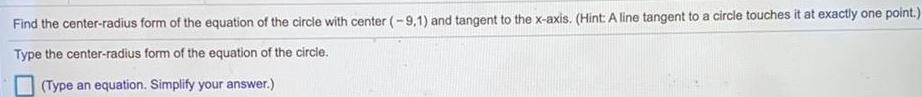Math
Circle
Find the center radius form of the equation of the circle with center 9 1 and tangent to the x axis Hint A line tangent to a circle touches it at exactly one point Type the center radius form of the equation of the circle Type an equation Simplify your answer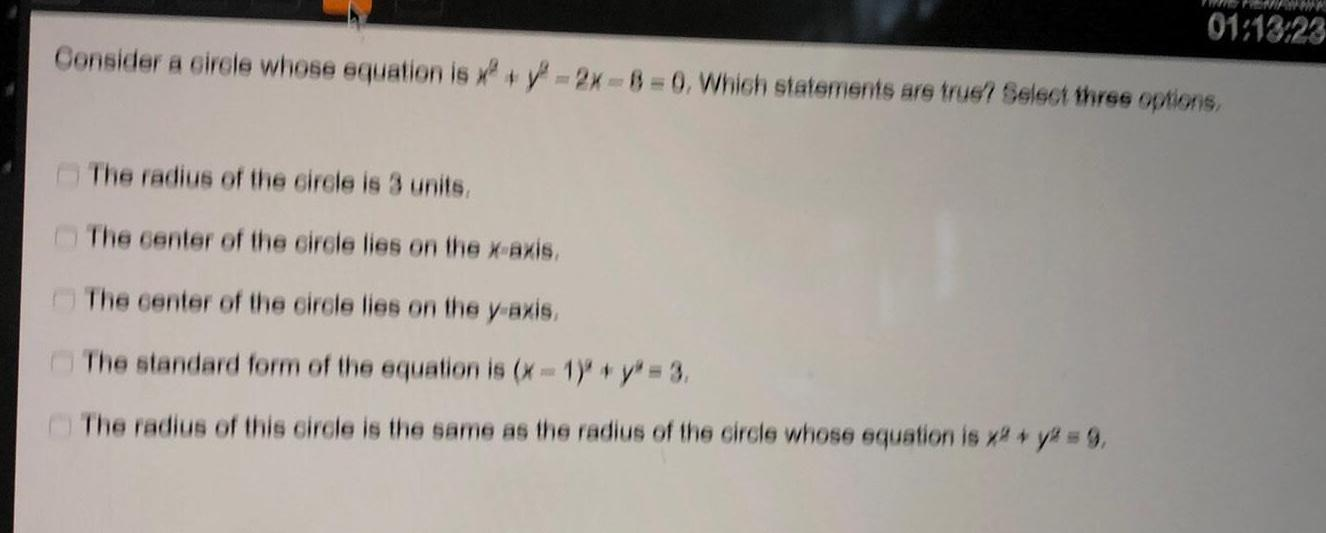Math
Circle
Consider a circle whose equation is x y 2x 8 0 Which statements are true Select three options The radius of the circle is 3 units The center of the circle lies on the x axis The center of the circle lies on the y axis The standard form of the equation is x 1 y 3 The radius of this circle is the same as the radius of the circle whose equation is x y 9 wwww 01 13 23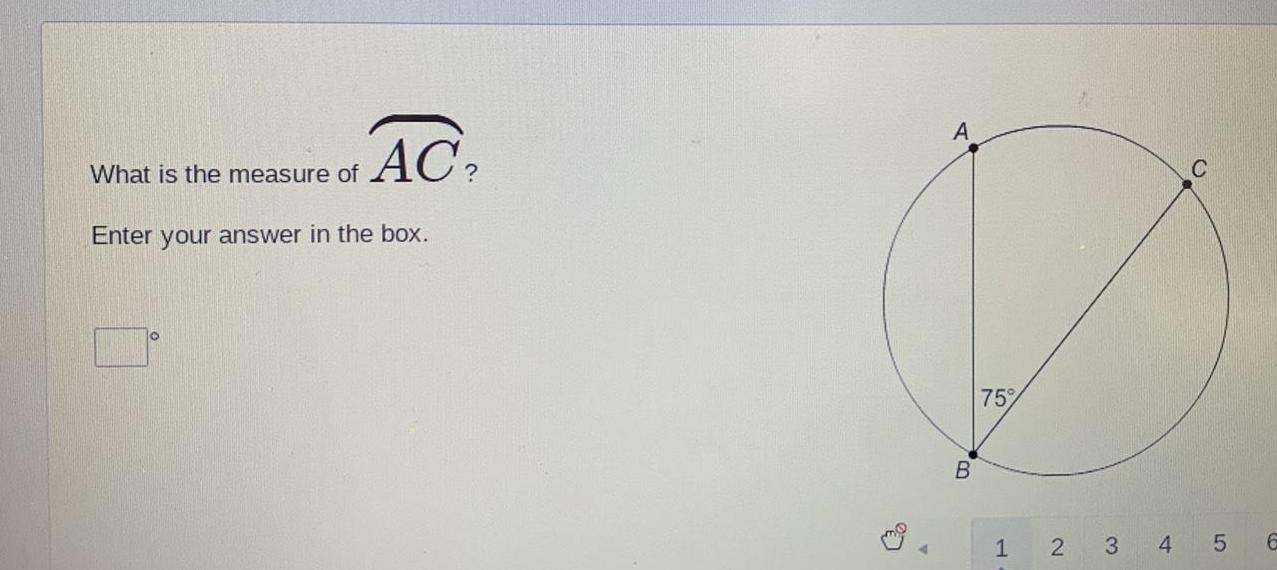Math
Circle
What is the measure of AC Enter your answer in the box A B 75 1 2 3 4 C 5 6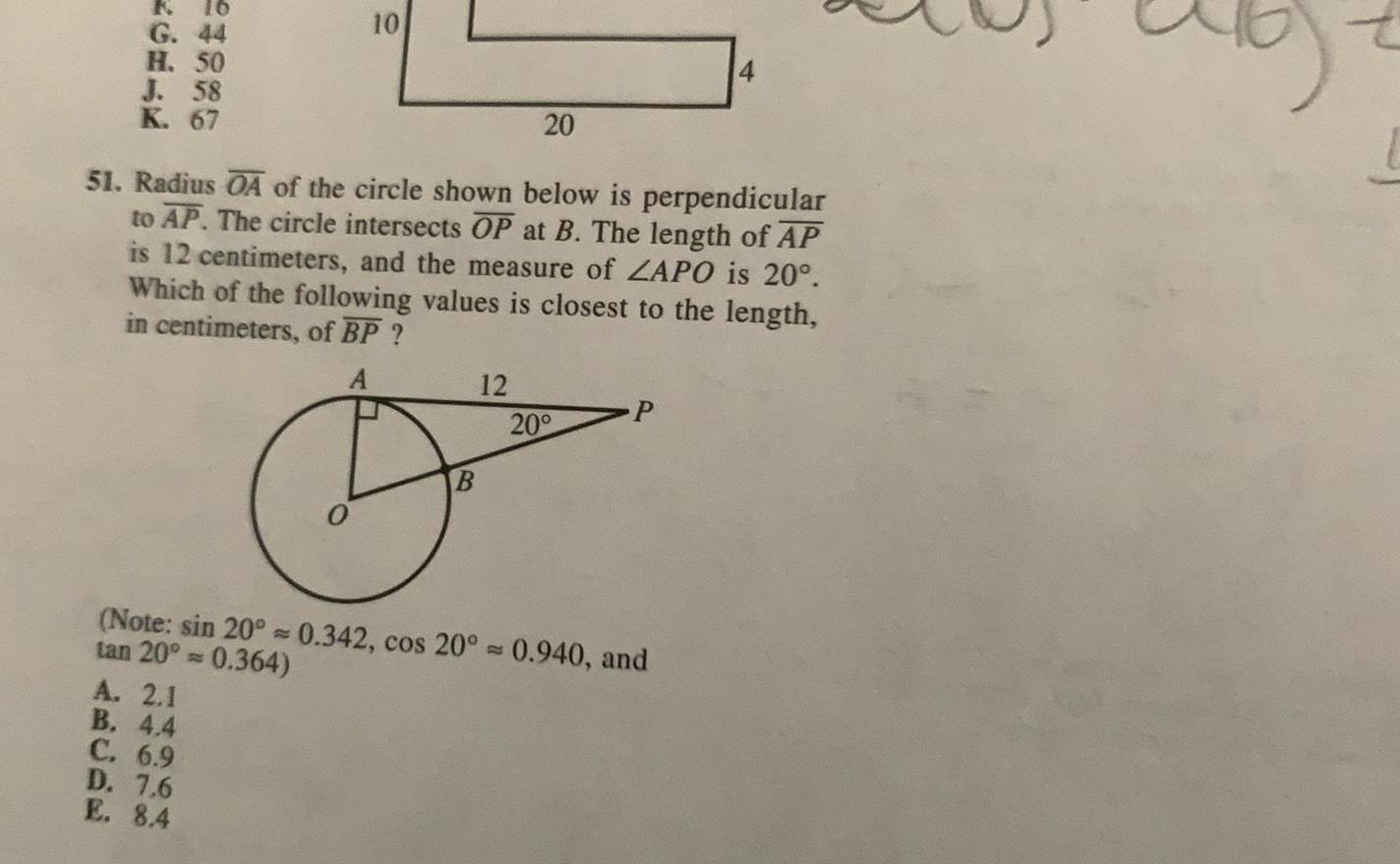Math
Circle
G 44 H 50 J 58 K 67 10 A 2 1 B 4 4 C 6 9 D 7 6 E 8 4 51 Radius OA of the circle shown below is perpendicular to AP The circle intersects OP at B The length of AP is 12 centimeters and the measure of ZAPO is 20 Which of the following values is closest to the length in centimeters of BP A B 20 12 20 P Note sin 20 0 342 cos 20 0 940 and tan 20 0 364 4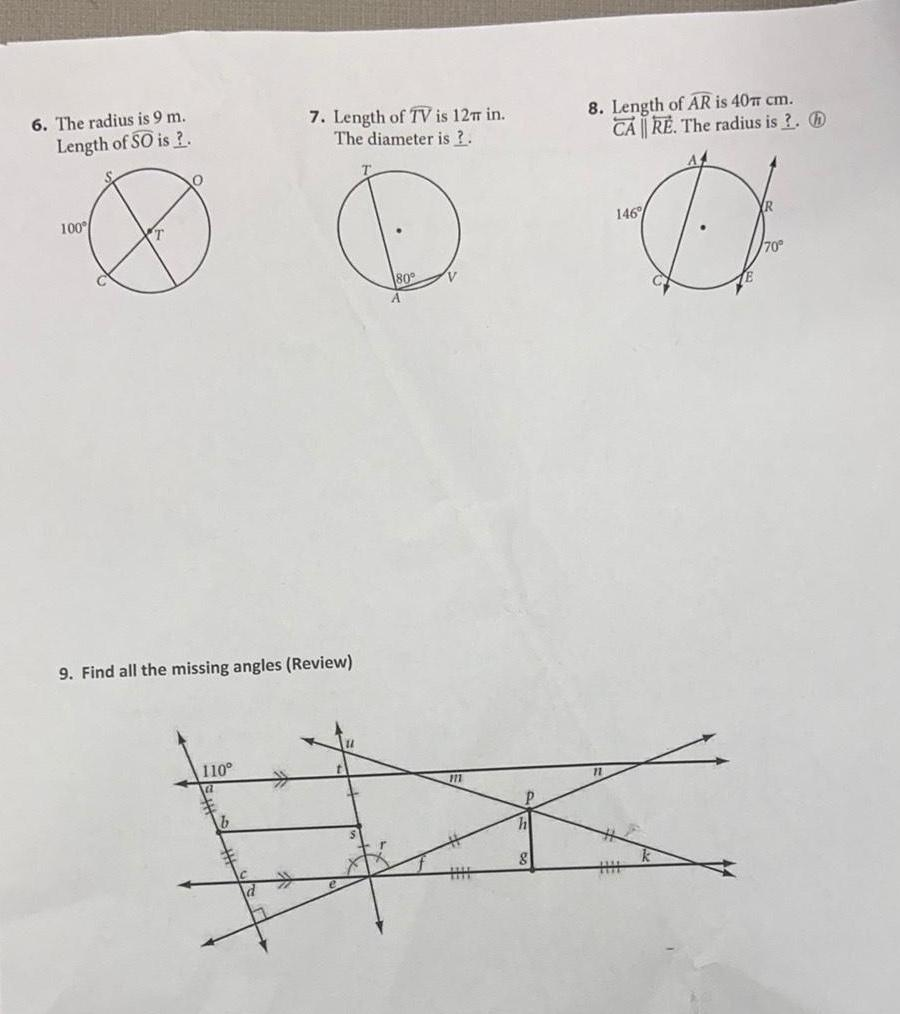Math
Circle
6 The radius is 9 m Length of SO is 100 T 7 Length of TV is 12 in The diameter is 9 Find all the missing angles Review 110 11 80 111 h 8 Length of AR is 40 cm CARE The radius is 8 146 D 70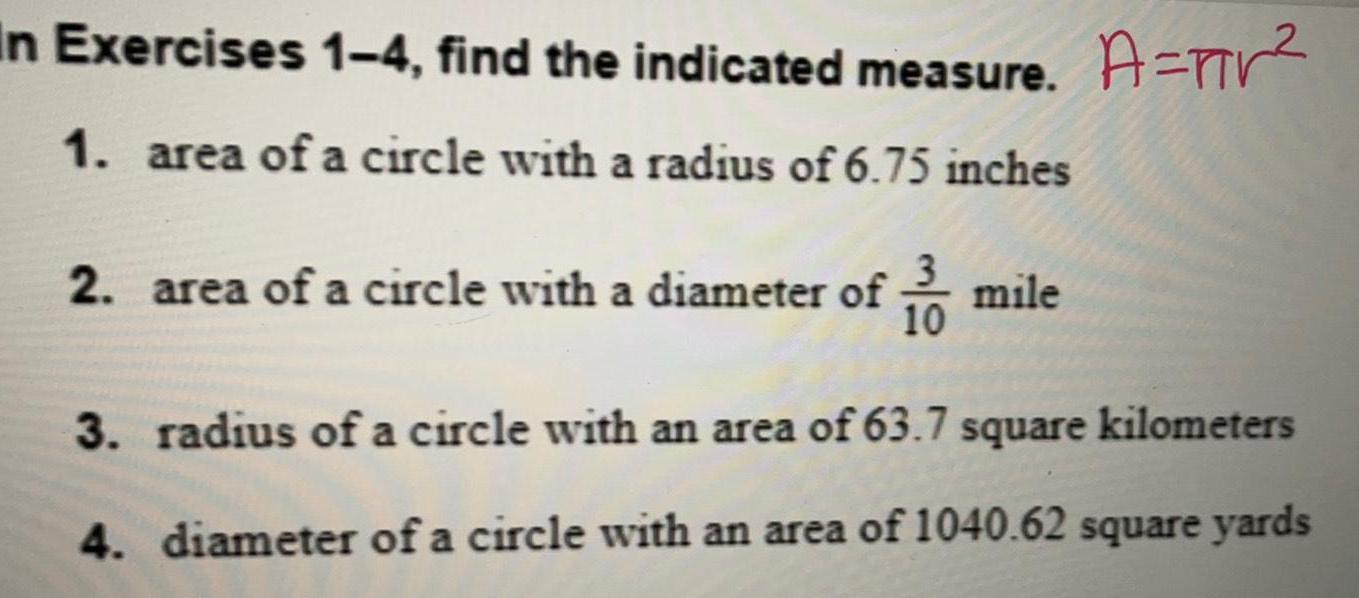Math
Circle
In Exercises 1 4 find the indicated measure A 1 area of a circle with a radius of 6 75 inches 2 area of a circle with a diameter of mile 10 3 radius of a circle with an area of 63 7 square kilometers 4 diameter of a circle with an area of 1040 62 square yards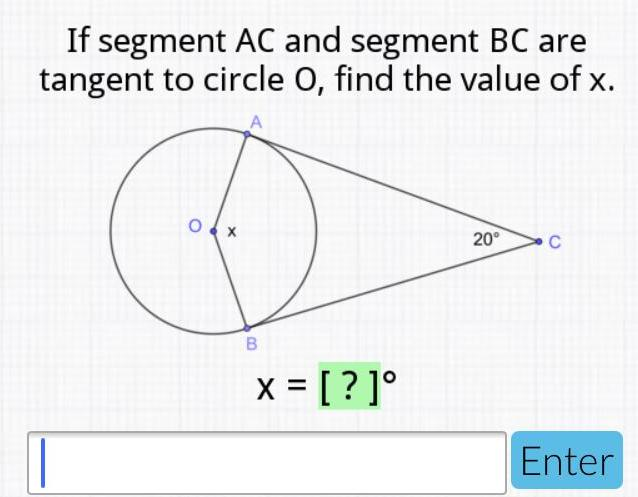Math
Circle
If segment AC and segment BC are tangent to circle O find the value of x 0 x B x 20 C Enter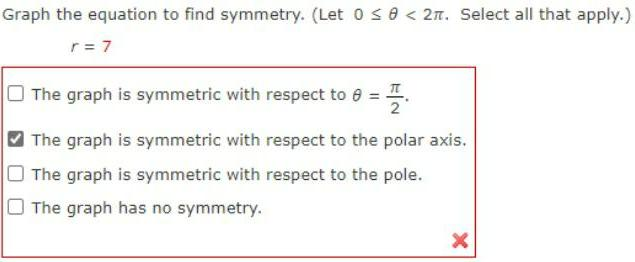Math
Circle
Graph the equation to find symmetry. (Let 0 ≤ θ< 2π. Select all that apply.) r = 7 The graph is symmetric with respect to θ = 2π The graph is symmetric with respect to the polar axis. The graph is symmetric with respect to the pole. The graph has no symmetry.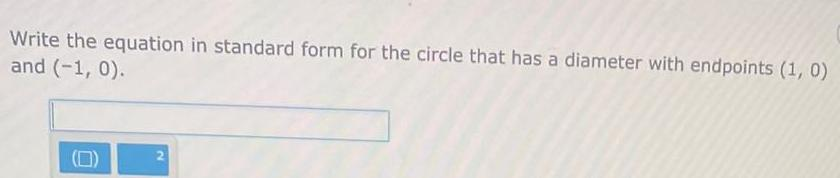Math
Circle
Write the equation in standard form for the circle that has a diameter with endpoints (1, 0) and (-1,0). (0)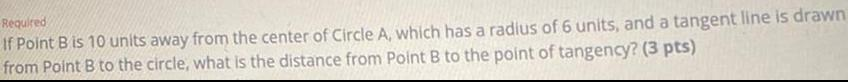Math
Circle
If Point B is 10 units away from the center of Circle A, which has a radius of 6 units, and a tangent line is drawn from Point B to the circle, what is the distance from Point B to the point of tangency?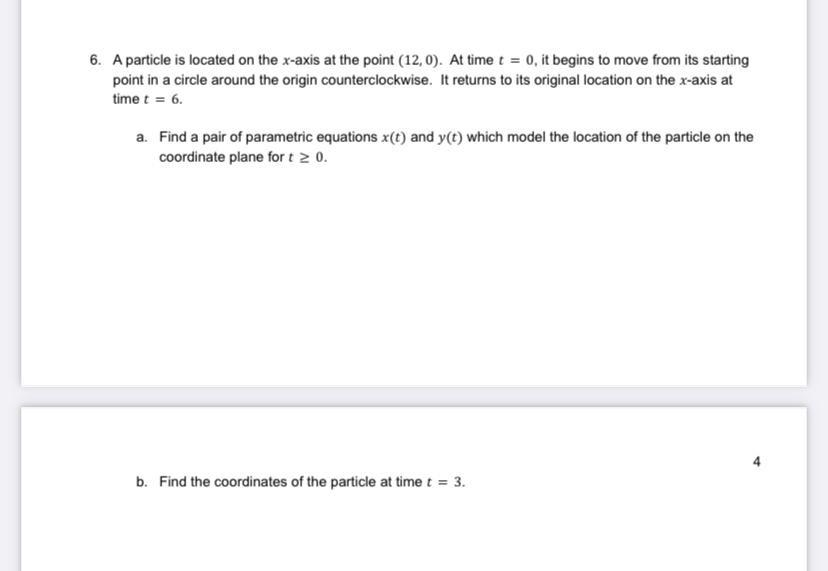Calculus
Circle
A particle is located on the x-axis at the point (12,0). At time t = 0, it begins to move from its starting point in a circle around the origin counterclockwise. It returns to its original location on the x-axis at time t = 6. a. Find a pair of parametric equations x(t) and y(t) which model the location of the particle on the coordinate plane for t≥0. b. Find the coordinates of the particle at time t = 3.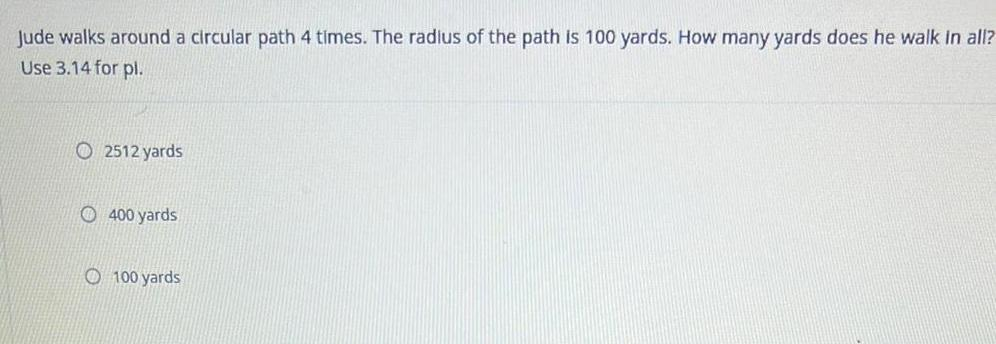Math
Circle
Jude walks around a circular path 4 times. The radius of the path is 100 yards. How many yards does he walk in all? Use 3.14 for pl. A. 2512 yards B. 400 yards C. 100 yards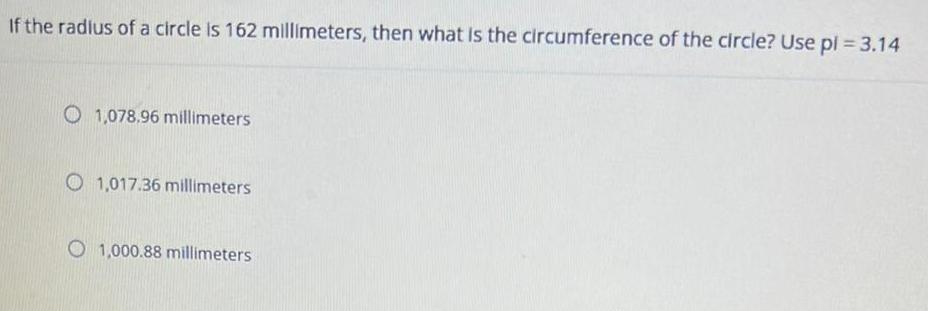Math
Circle
If the radius of a circle is 162 millimeters, then what is the circumference of the circle? Use pl = 3.14 A. 1,078.96 millimeters B. 1,017.36 millimeters C. 1,000.88 millimeters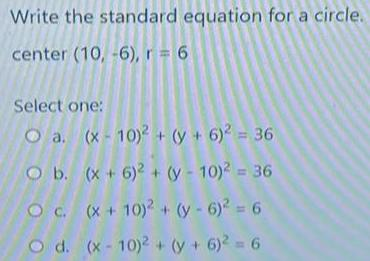Math
Circle
Write the standard equation for a circle. center (10, -6), r = 6 Select one: a. (x-10)² + (y + 6)² = 36 b. (x + 6)² + (y - 10)² = 36 c.(x + 10)² + (y - 6)² = 6 d. (x-10)² + (y + 6)² = 6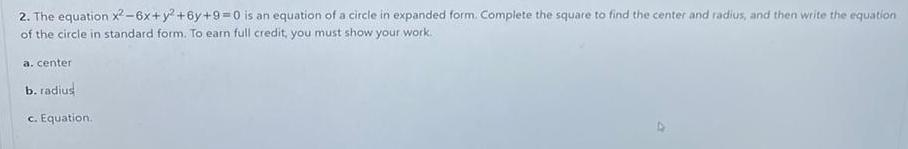Math
Circle
The equation x²-6x + y² +6y+9=0 is an equation of a circle in expanded form. Complete the square to find the center and radius, and then write the equation of the circle in standard form. To earn full credit, you must show your work. a. center b. radius c. Equation..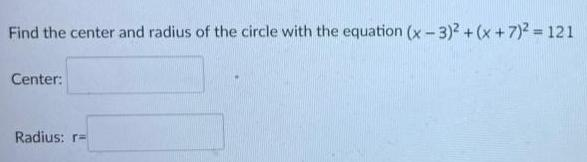Math
Circle
Find the center and radius of the circle with the equation (x-3)² + (x+7)² = 121 Center: Radius: r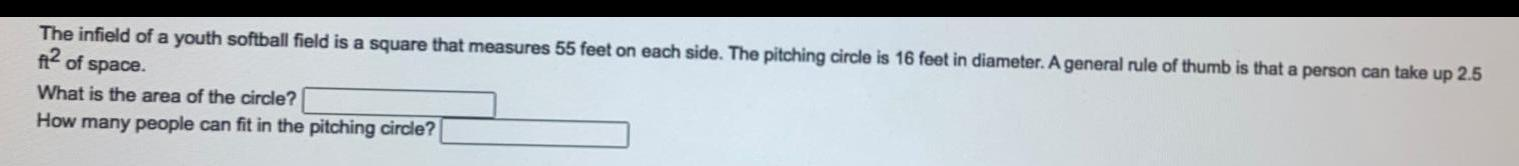Math
Circle
The infield of a youth softball field is a square that measures 55 feet on each side. The pitching circle is 16 feet in diameter. A general rule of thumb is that a person can take up 2.5 ft² of space. What is the area of the circle? How many people can fit in the pitching circle?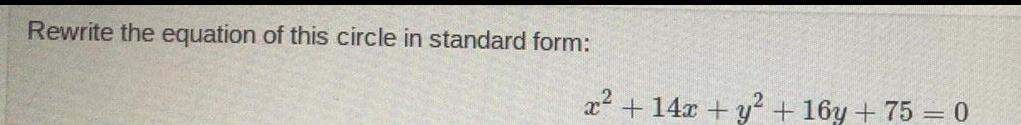Math
Circle
Rewrite the equation of this circle in standard form: x² + 14x + y² + 16y + 75 = 0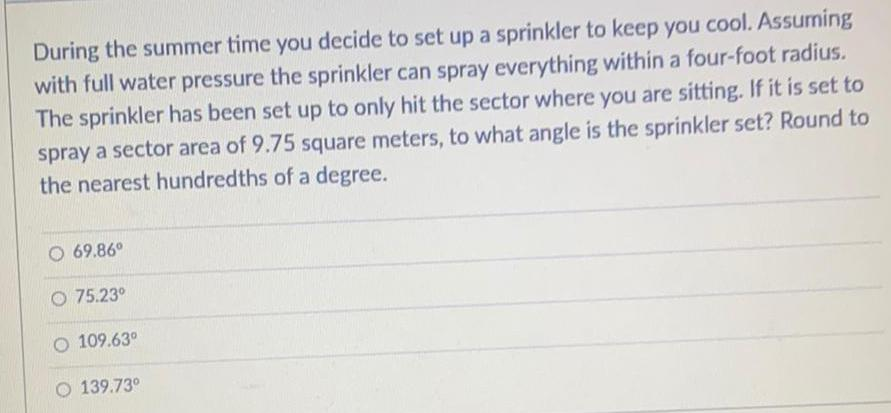Math
Circle
During the summer time you decide to set up a sprinkler to keep you cool. Assuming with full water pressure the sprinkler can spray everything within a four-foot radius. The sprinkler has been set up to only hit the sector where you are sitting. If it is set to spray a sector area of 9.75 square meters, to what angle is the sprinkler set? Round to the nearest hundredths of a degree. 69.86⁰ 75.23⁰ 109.63⁰ 139.73°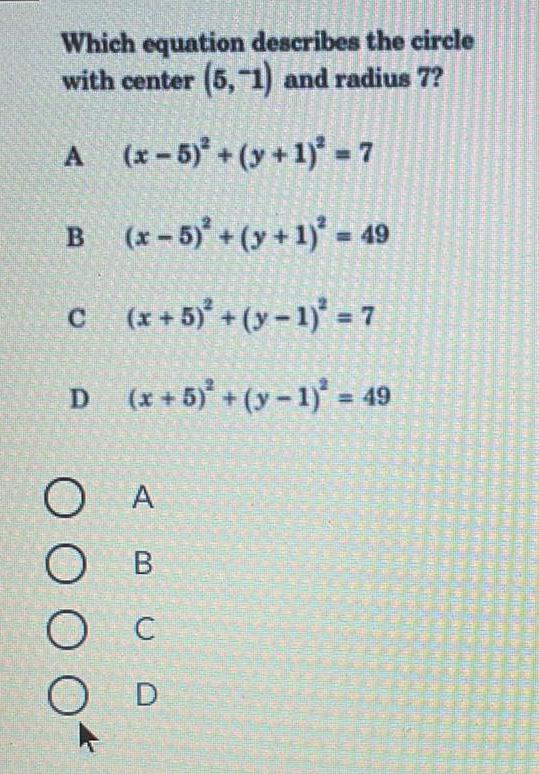Math
Circle
Which equation describes the circle with center (5,-1) and radius 7? A (x-5)² +(y + 1)² = 7 B (x-5)² +(y+1)² = 49 C (x + 5)²+(y-1)³ = 7 D (x+5)² +(y-1)² = 49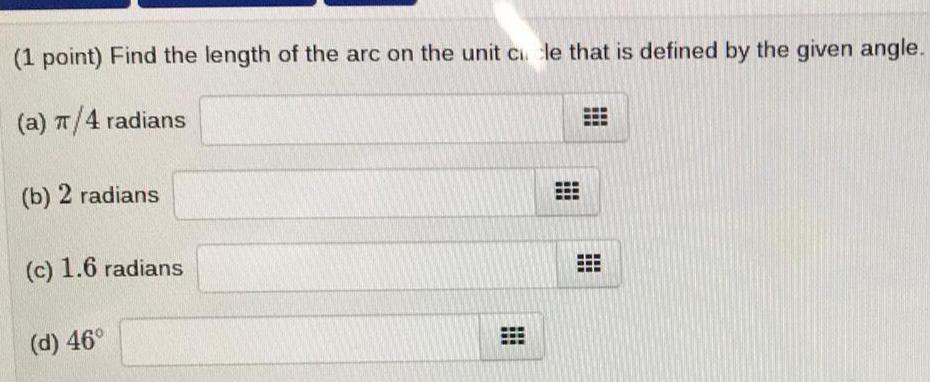Math
Circle
(1 point) Find the length of the arc on the unit cicle that is defined by the given angle. (a) π/4 radians (b) 2 radians (c) 1.6 radians (d) 46°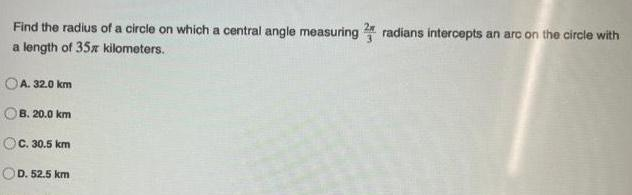Math
Circle
Find the radius of a circle on which a central angle measuring 2 radians intercepts an arc on the circle with a length of 35% kilometers. A. 32.0 km B. 20.0 km C. 30.5 km D. 52.5 km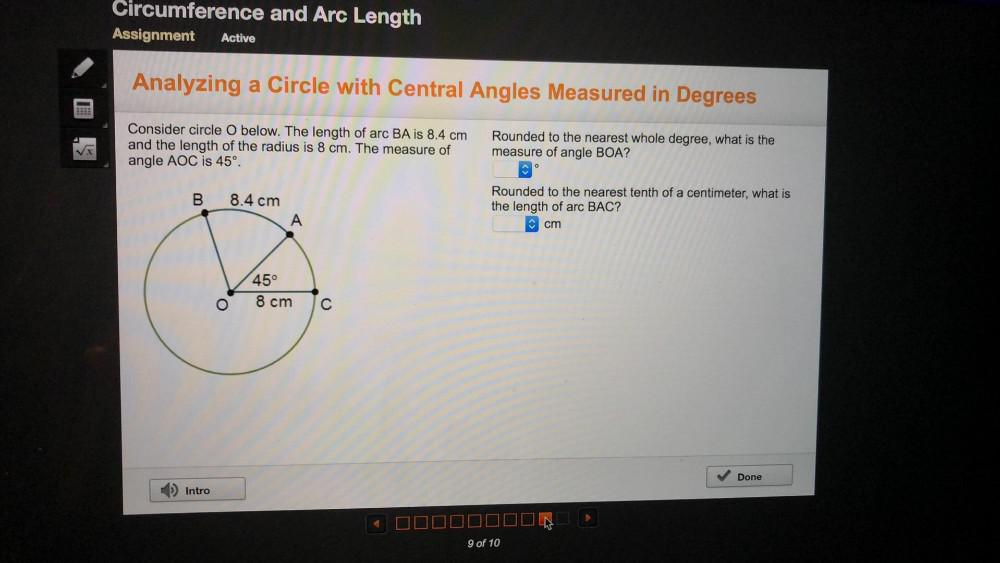Math
Circle
Consider circle O below. The length of arc BA is 8.4 cm and the length of the radius is 8 cm. The measure of angle AOC is 45°. Rounded to the nearest whole degree, what is the measure of angle BOA? Rounded to the nearest tenth of a centimeter, what is the length of arc BAC?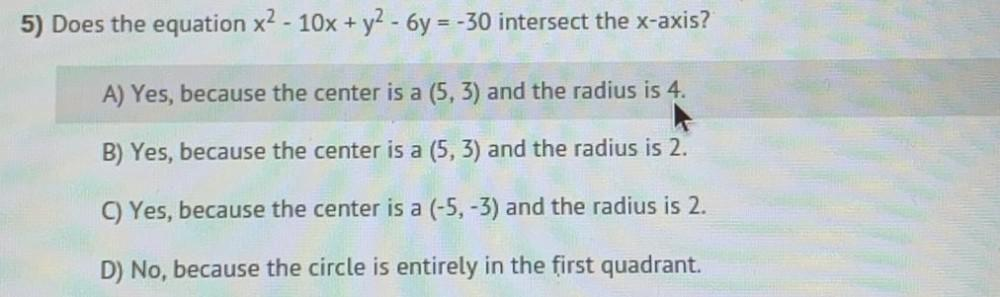Math
Circle
5) Does the equation x² - 10x + y² - 6y = -30 intersect the x-axis? A) Yes, because the center is a (5, 3) and the radius is 4. B) Yes, because the center is a (5, 3) and the radius is 2. C) Yes, because the center is a (-5, -3) and the radius is 2. D) No, because the circle is entirely in the first quadrant.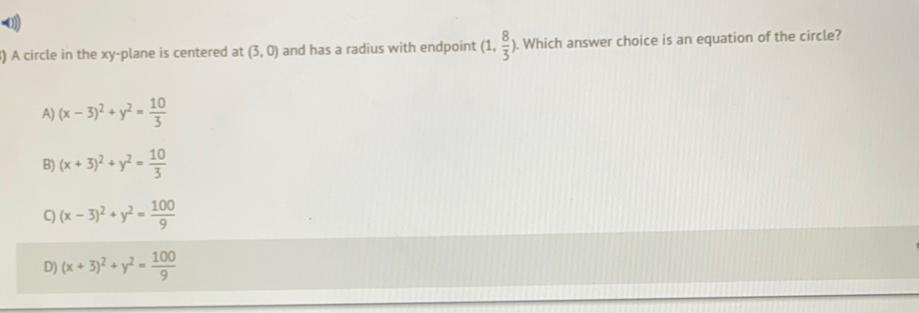Math
Circle
A circle in the xy-plane is centered at (3, 0) and has a radius with endpoint (1, 8/3). Which answer choice is an equation of the circle? A) (x – 3)^2 + y^2 = 10/3 B) (x + 3)^2 + y^2 =10/3 C)(x - 3)^2 + y^2 =100/9 D) (x + 3)^2 + y^2 - 100/9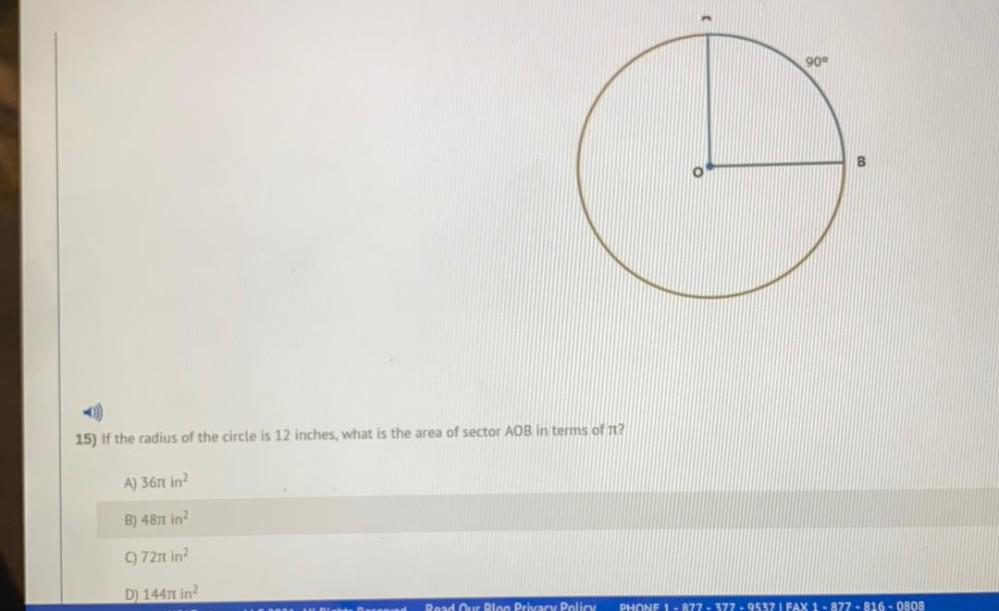Math
Circle
if the radius of the circle is 12 inches, what is the area of sector AOB in terms of it? given; central angle ,AOB= 90 A) 367 π in^2 B) 487 π in^2 (C)721 π in^2 D) 14471 π in^2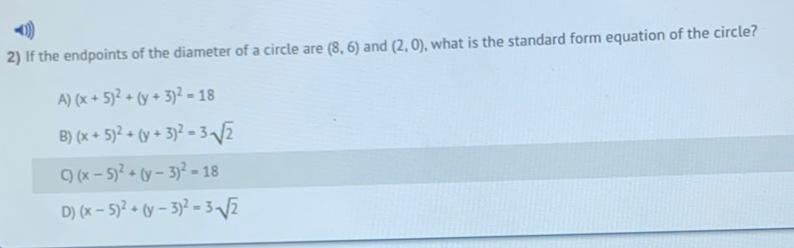Math
Circle
If the endpoints of the diameter of a circle are (8,6) and (2.0), what is the standard form equation of the circle? A) (x + 5)^2 + (y + 3)^2 =18 B) (x + 5)^2 + (y + 3)^2 = 3√2 c)(x - 5)^2 + (y - 3)^2 =18 D)(x - 5)^2 + (y - 3)^2 = 3√2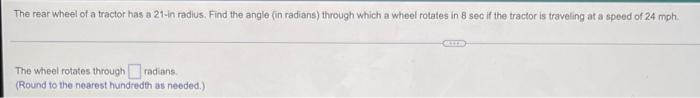Math
Circle
The rear wheel of a tractor has a 21-in radius. Find the angle (in radians) through which a wheel rotates in 8 sec if the tractor is traveling at a speed of 24 mph, The wheel rotates through_____radians.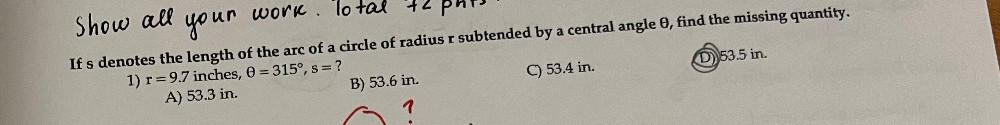Math
Circle
If s denotes the length of the arc of a circle of radius r subtended by a central angle θ, find the missing quantity. 1) r=9.7 inches, θ = 315º, s = ? A) 53.3 in. B) 53.6 in. C) 53.4 in. D) 53.5 in.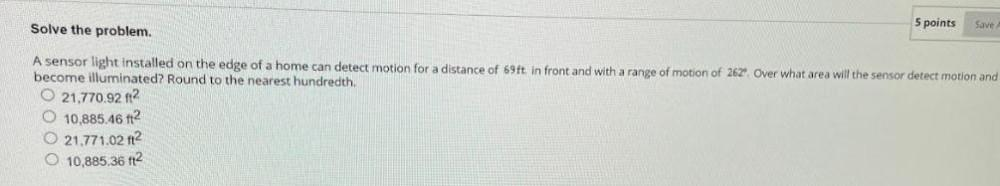Math
Circle
A sensor light installed on the edge of a home can detect motion for a distance of 69ft in front and with a range of motion of 262° Over what area will the sensor detect motion and become illuminated? Round to the nearest hundredth. 21,770.92 ft2. 10,885.46 ft2. 21,771.02 ft2. 10,885.36 ft2.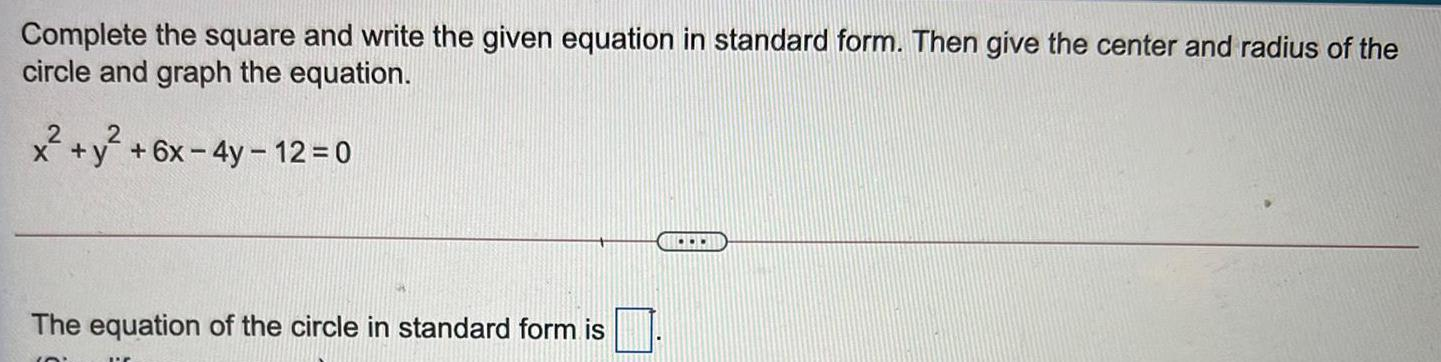Math
Circle
Complete the square and write the given equation in standard form. Then give the center and radius of the circle and graph the equation. x² + y² +6x-4y - 12 = 0 The equation of the circle in standard form is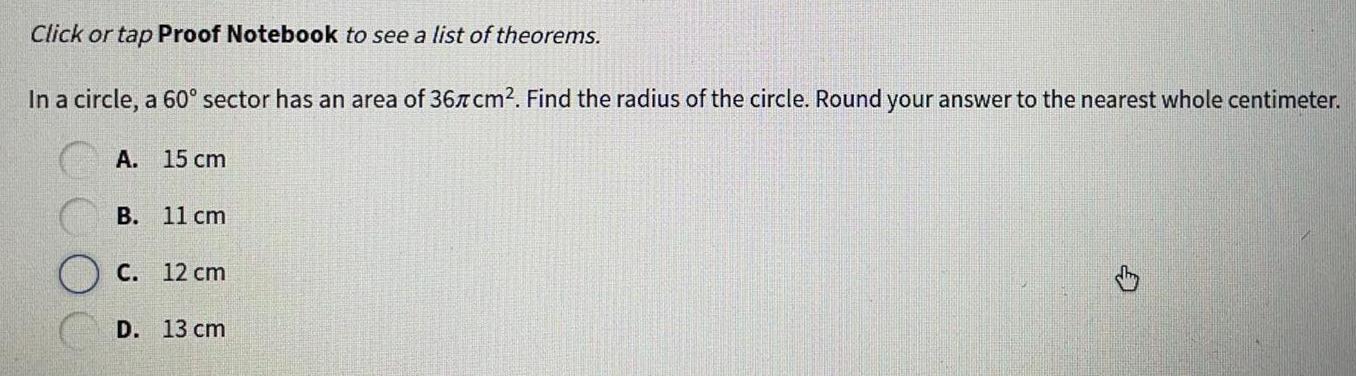Math
Circle
Click or tap Proof Notebook to see a list of theorems. In a circle, a 60° sector has an area of 36π cm². Find the radius of the circle. Round your answer to the nearest whole centimeter. A.15 cm B.11 cm C.12 cm D.13 cm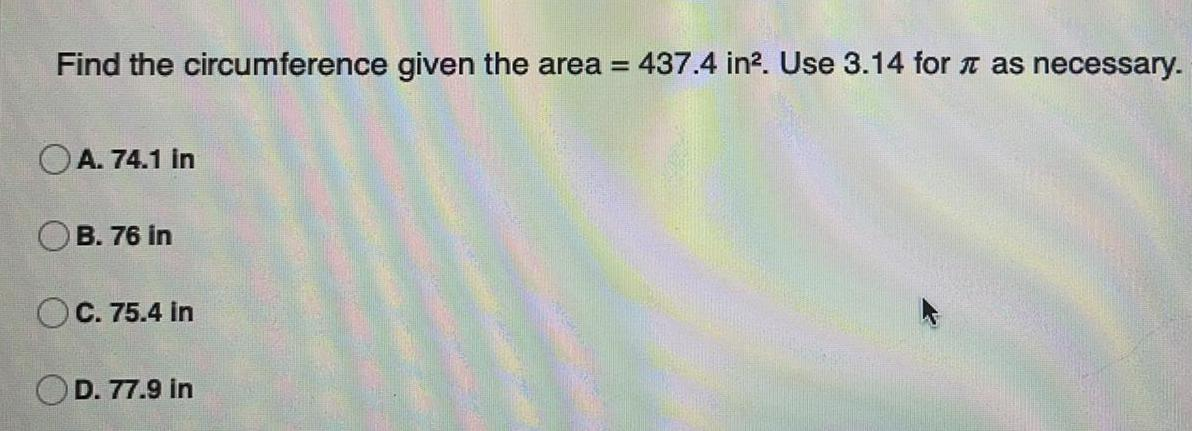Math
Circle
Find the circumference given the area =437.4 in². Use 3.14 for π as necessary. A. 74.1 in B. 76 in C. 75.4 in D. 77.9 in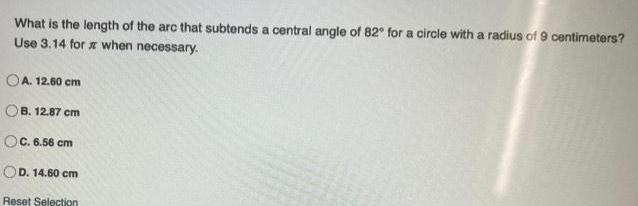Math
Circle
What is the length of the arc that subtends a central angle of 82° for a circle with a radius of 9 centimeters? Use 3.14 for π when necessary. A. 12.60 cm B. 12.87 cm C. 6.56 cm D. 14.60 cm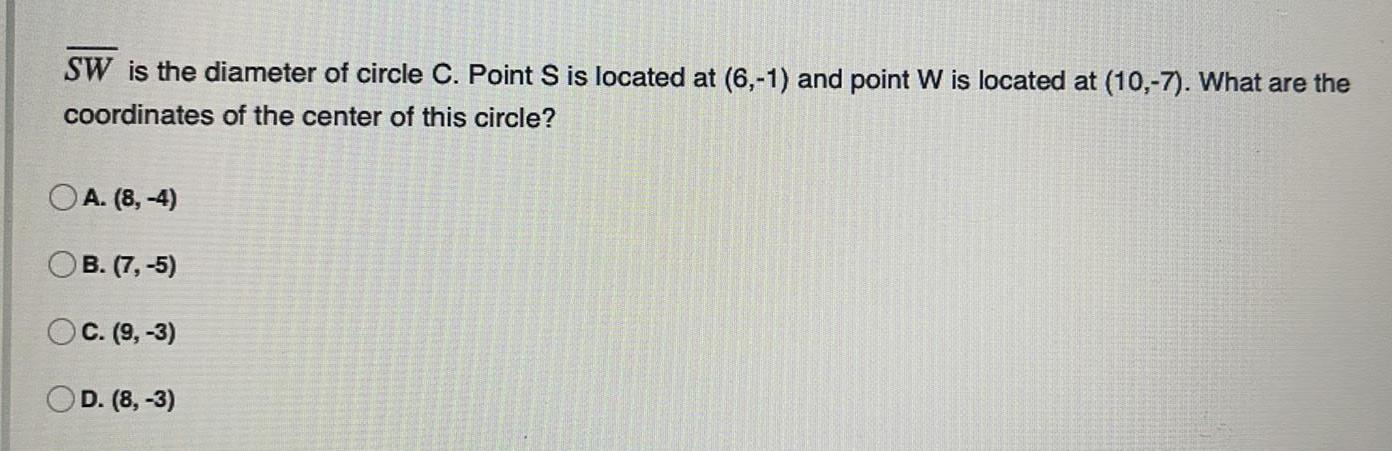Math
Circle
SW is the diameter of circle C. Point S is located at (6,-1) and point W is located at (10,-7). What are the coordinates of the center of this circle? A. (8,-4) B. (7,-5) C. (9,-3) D. (8, -3)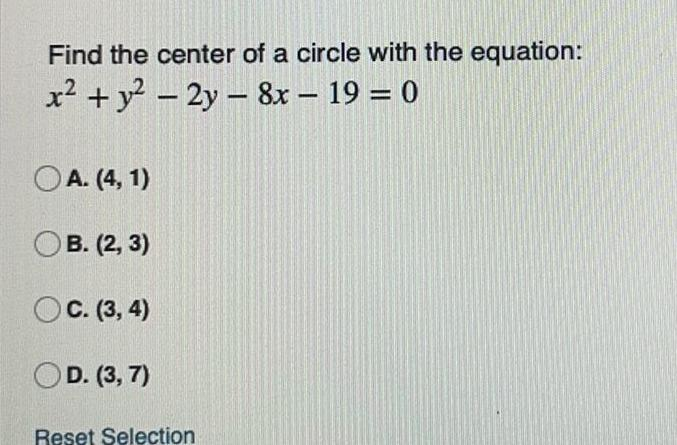Math
Circle
Find the center of a circle with the equation: x² + y² - 2y - 8x - 19 = 0 A. (4,1) B. (2, 3) C. (3, 4) D. (3,7)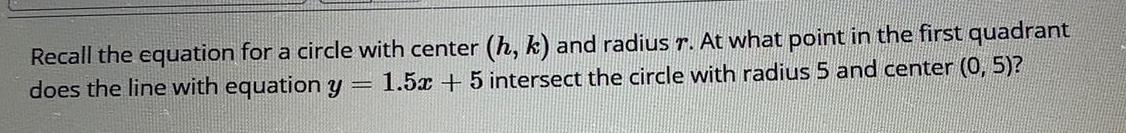Math
Circle
Recall the equation for a circle with center (h, k) and radius r. At what point in the first quadrant does the line with equation y=1.5x+5 intersect the circle with radius 5 and center (0, 5)?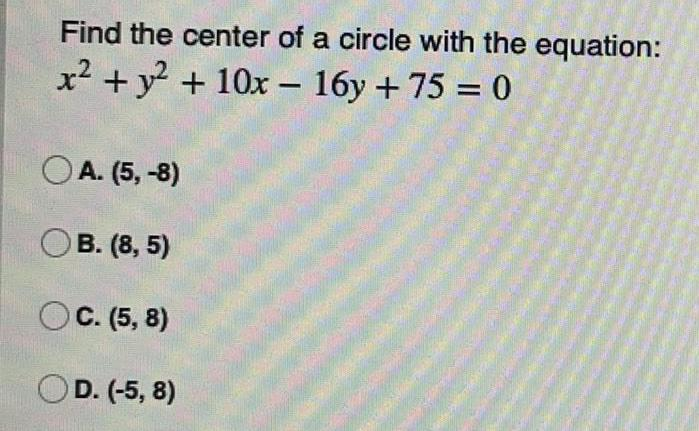Math
Circle
Find the center of a circle with the equation: x² + y² + 10x - 16y + 75 = 0 A. (5,-8) B. (8,5) C. (5,8) D. (-5, 8)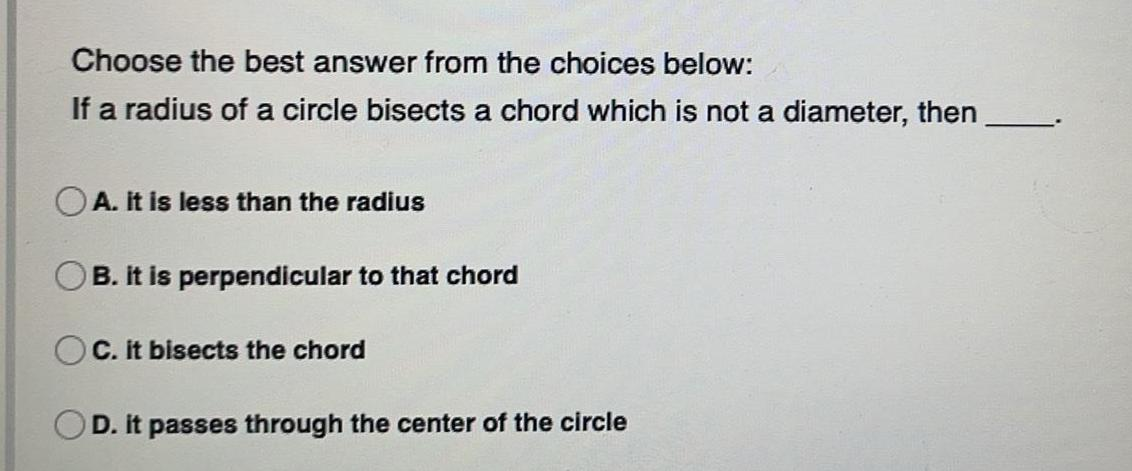Math
Circle
Choose the best answer from the choices below: If a radius of a circle bisects a chord which is not a diameter, then A. it is less than the radius B. it is perpendicular to that chord C. it bisects the chord D. it passes through the center of the circle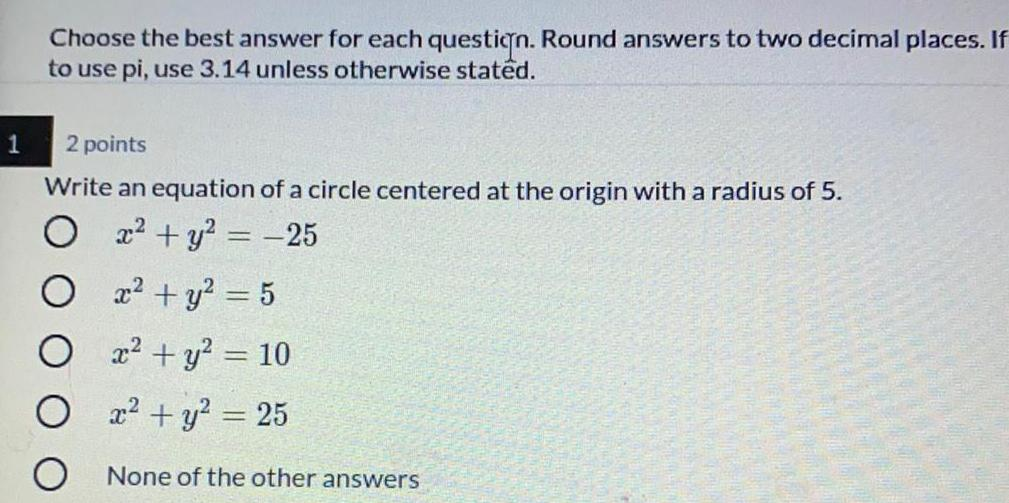Math
Circle
Choose the best answer for each question. Round answers to two decimal places. If to use pi, use 3.14 unless otherwise stated. Write an equation of a circle centered at the origin with a radius of 5. x² + y² = -25 x² + y² = 5 x² + y² = 10 x² + y² = 25 None of the other answers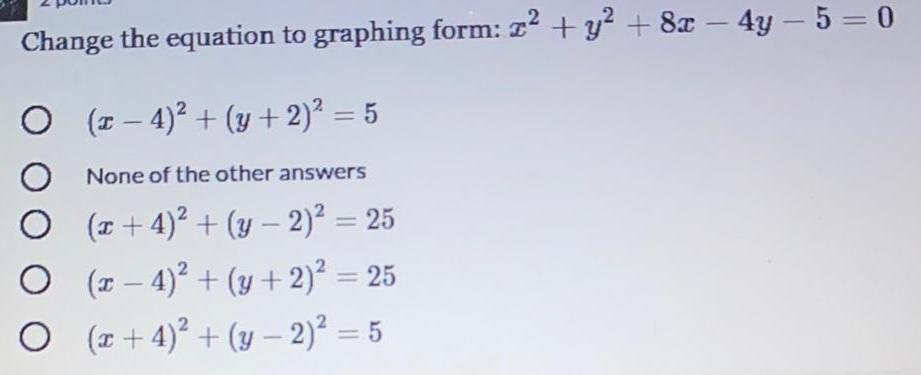Math
Circle
Change the equation to graphing form: x² + y² + 8x - 4y - 5 = 0 (x-4)² + (y + 2)² = 5 None of the other answers (x+4)² + (y − 2)² = 25 (x-4)² + (y + 2)² = 25 (x+4)² + (y− 2)² = 5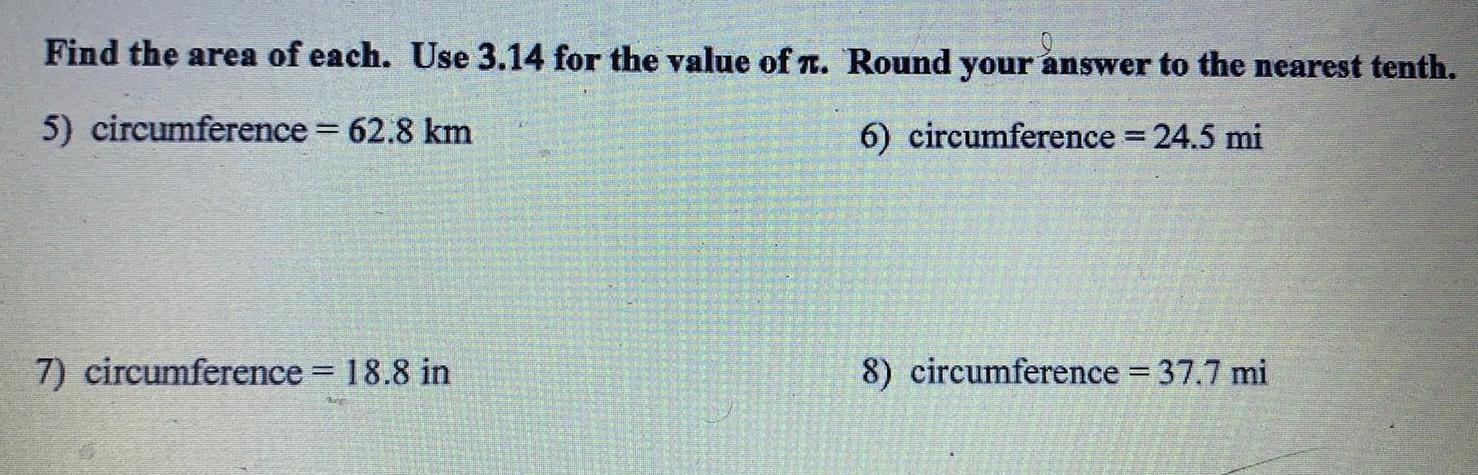Math
Circle
Find the area of each. Use 3.14 for the value of л. Round your answer to the nearest tenth. 5) circumference = 62.8 km 6) circumference = 24.5 mi 7) circumference = 18.8 in 8) circumference = 37.7 mi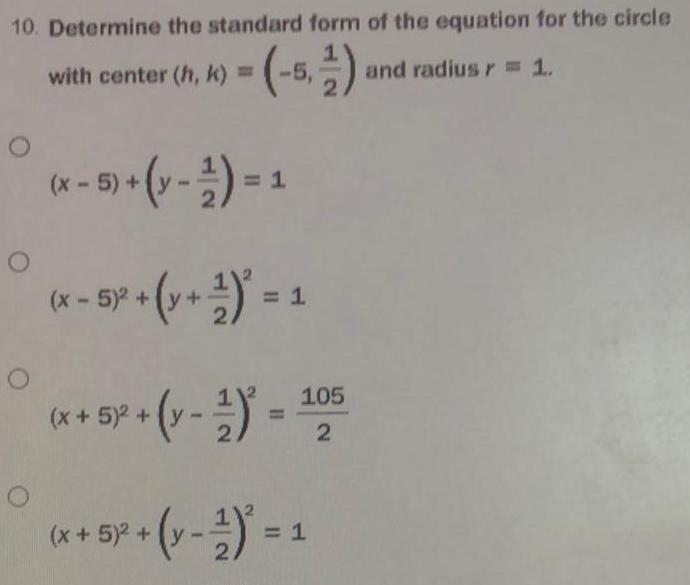Math
Circle
Determine the standard form of the equation for the circle with center (h, k) = (-5,1/2) and radius r = 1.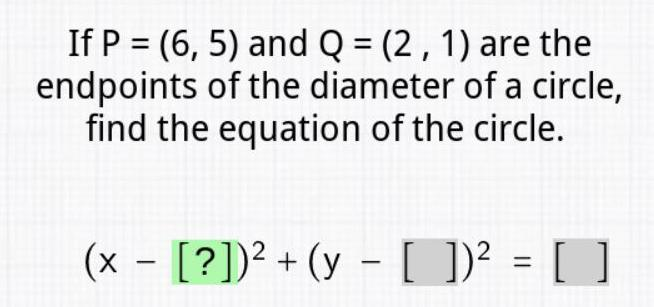Math
Circle
If P = (6, 5) and Q = (2, 1) are the endpoints of the diameter of a circle, find the equation of the circle.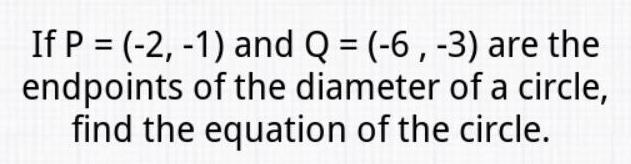Math
Circle
If P = (-2, -1) and Q = (-6, -3) are the endpoints of the diameter of a circle, find the equation of the circle.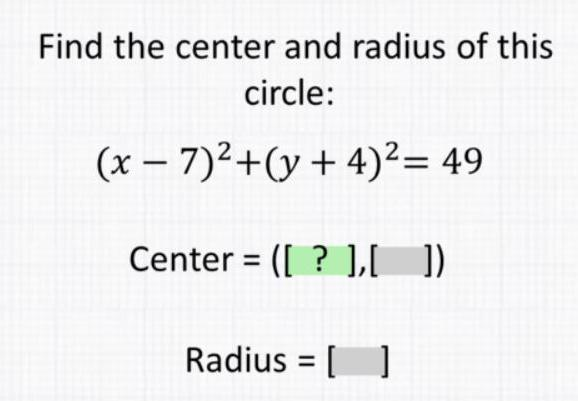Math
Circle
Find the center and radius of this circle: (x-7)²+(y + 4)² = 49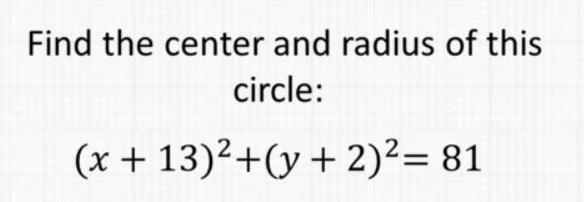Math
Circle
Find the center and radius of this circle: (x + 13)2+(y + 2)² = 81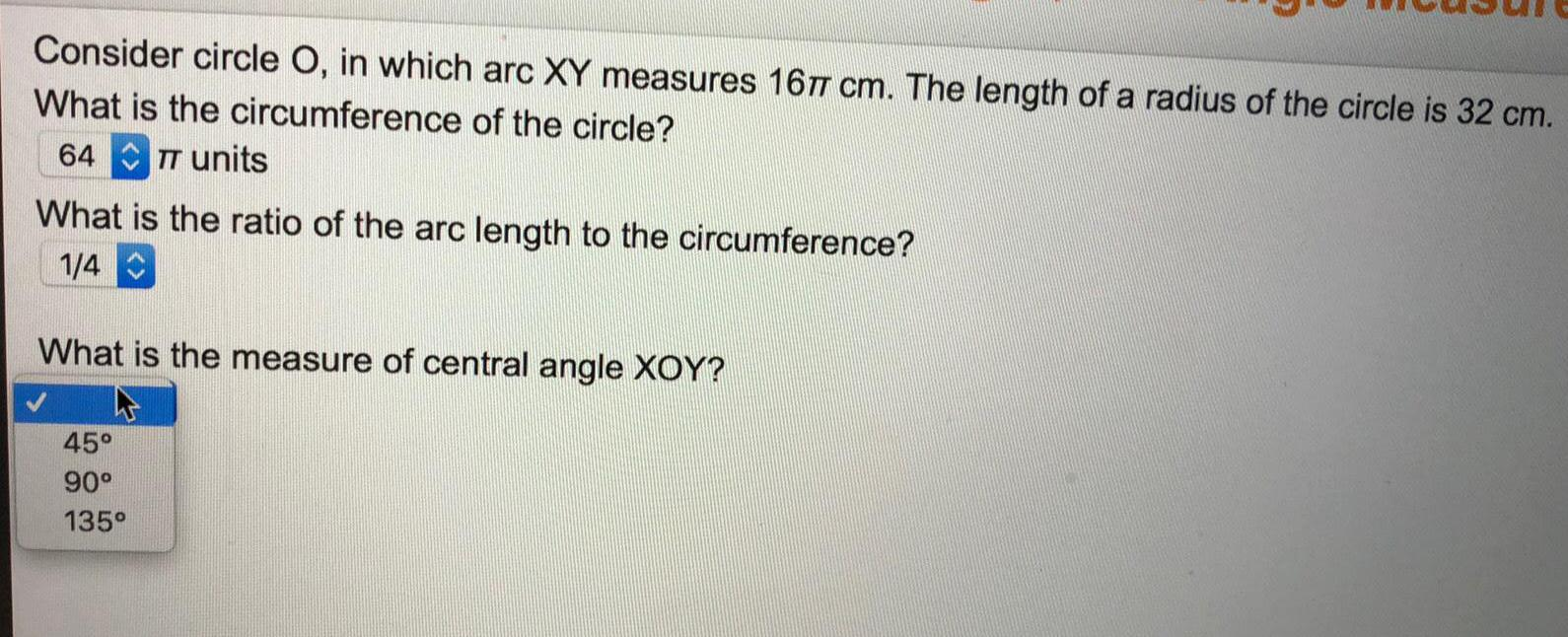Math
Circle
Consider circle O, in which arc XY measures 16π cm. The length of a radius of the circle is 32 cm. What is the circumference of the circle? π units What is the ratio of the arc length to the circumference? What is the measure of central angle XOY? 45° 90° 135°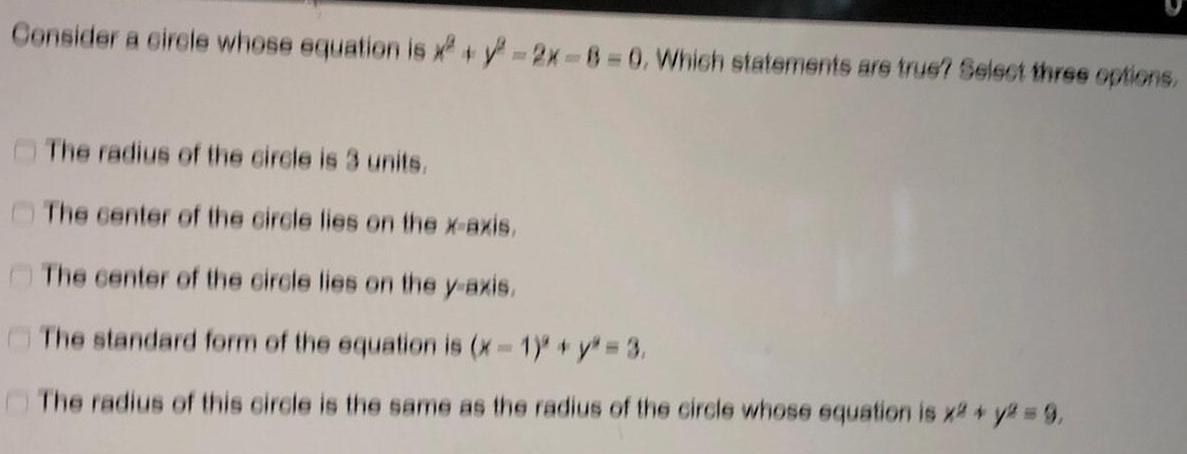Math
Circle
Consider a circle whose equation is x² + y² =2x-8-0. Which statements are true? Select three options. The radius of the circle is 3 units. The center of the circle lies on the x-axis. The center of the circle lies on the y-axis. The standard form of the equation is (x - 1)² + y² = 3. The radius of this circle is the same as the radius of the circle whose equation is x² + y² = 9,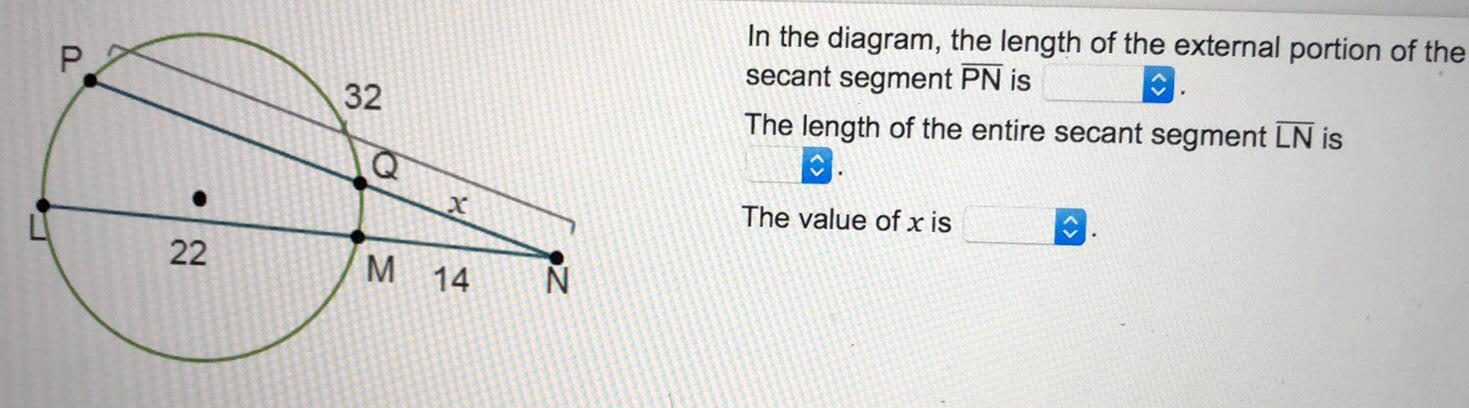Math
Circle
In the diagram, the length of the external portion of the secant segment PN is The length of the entire secant segment LN is The value of x is